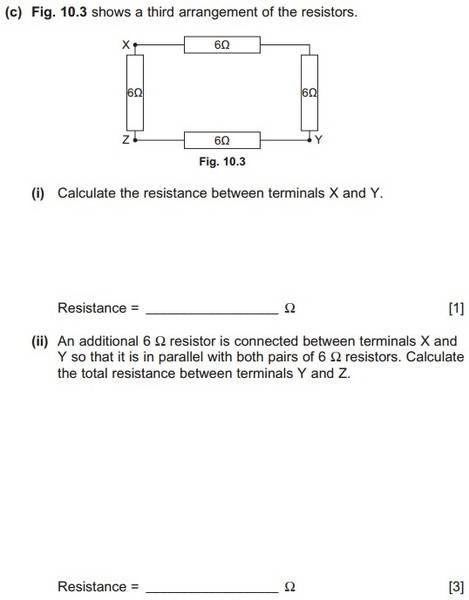# Calculating the resistance between 2 terminals of circuit

• TiernanW

## Homework Statement## Homework Equations

1/Rt = 1/R1 + 1/R2 + ...

## The Attempt at a Solution

I can do part (i) okay. It is simply 1/12 + 1/12 = 1/6, so R = 6. But I have been trying for ages to figure out part (ii). I tried re-drawing the circuit in different forms but it didn't help me.

The one that makes most sense to me is 1/12 + 1/12 + 1/6 which gives the total resistance as 3, but the correct answer is 3.75, and I seriously can't figure out why no matter how hard I try.

Show us your drawing. Label the resistors A,B,C,D etc

•TiernanW
Equivalent resistance is asked between Y and Z. Which resistances are in series and in parallel when viewed from terminals Y and Z?

•TiernanW
Why do you figure these things out as soon as you post them? I was honestly at this for ages and just got it.

Here is my solution for anyone viewing this in the future:
http://i.imgur.com/nnF8t83.jpg

Hey TiernanW,

Try this:

1. As stated in part (ii), draw a new wire with a new resistor directly between terminals X and Y (along an imaginary diagonal through the middle of the diagram).
2. Redraw the diagram so that all resistors are oriented in the same direction, so they actually sit parallel to one another in the diagram.
3. Simplify using the techniques you already know, beginning with summing the resistors in series, and then solving for the three equivalent parallel resistors.

Alternately, you can redraw the original diagram like this, and then add the new resistor into a parallel configuration with the simplified original - whichever you find easiest.

I hope this helps - I always found it easier to simplify circuits when all components are arranged this way.

Best,

Richard

Why do you figure these things out as soon as you post them? I was honestly at this for ages and just got it.

Here is my solution for anyone viewing this in the future:
http://i.imgur.com/nnF8t83.jpg
Looks good!

Why isn't it just 6 ohms? 2 6 ohm resistors in series =12 ohms and another set of 12 ohms and the two in parallel. Looks like it should be 6 ohms. Sorry, that would be between X and Y. Between X and Z would be a 6 ohm resistor in parallel with an 18 ohm resistor. That would be 6 times 18 divided by 6 PLUS 18 which equals 4.5 ohms. Oops, that would be without the extra resistor.

Last edited:
Why isn't it just 6 ohms? 2 6 ohm resistors in series =12 ohms and another set of 12 ohms and the two in parallel. Looks like it should be 6 ohms.
That is for question 1. In question 2, equivalent resistance is asked between Y and Z.
Edit: I see you've already edited the post..

Why do you figure these things out as soon as you post them?
Because when you sort out your thoughts enought to explain the details you have explained it to yourself.

Part (ii) does not make sense as written.

If a 6Ω resistor is connected between points X and Y, then it is in parallel with the two pairs of 6Ω resistors IF we are considering resistance between points X and Y.

However, if we are considering resistance between points Y and Z, then the 6Ω resistor connected between points X and Y is in parallel with only one pair of the initial 6Ω resistors.